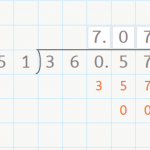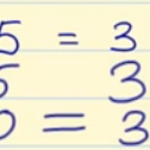Smartick is an online platform for children to master math in only 15 minutes a day

# I want to learn about: Operations & Algebraic Thinking

Learn operations and algebraic thinking in elementary with Smartick. Exercises, theory, tutorials, and teaching resources about operations and algebraic thinking in elementary aged children. Here you will find posts about operations and algebraic thinking to make learning mathematics easier and more fun.

Jun10

## Dividing a Decimal by a Whole NumberDividing a Decimal by a Whole Number Hello everyone! In this week’s post, we are going to talk about dividing a decimal by a whole number. You already know how to divide a whole number by a whole number by now, right? If not, check out our posts about dividing by a one-digit number here, […]

Jun09

## Properties of MultiplicationThe properties of multiplication are distributive, commutative, associative, removing a common factor and the neutral element. We are dedicating this post to examining the properties of multiplication, which are the following: Distributive property: The multiplication of a number by a sum is equal to the sum of the multiplications of this number by each one […]